## Example Questions

### Example Question #41 : Coordinate Geometry

Find the point where the line y = .25(x – 20) + 12 crosses the x-axis.

(–7,0)

(0,0)

(–28,0)

(12,0)

(0,–28)

(–28,0)

Explanation:

When the line crosses the x-axis, the y-coordinate is 0. Substitute 0 into the equation for y and solve for x.

.25(x – 20) + 12 = 0

.25x – 5 = –12

.25x = –7

x = –28

The answer is the point (–28,0).

### Example Question #2 : How To Find Out If A Point Is On A Line With An Equation

On a coordinate plane, two lines are represented by the equations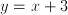and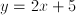. These two lines intersect at point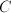. What are the coordinates of point?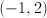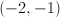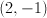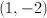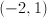Explanation:

You can solve for thewithin these two equations by eliminating the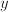. By doing this, you get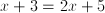.

Solve forto get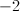and plugback into either equation to get the value ofas 1.

### Example Question #3 : How To Find Out If A Point Is On A Line With An Equation

If the two lines represented by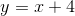and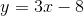intersect at point, what are the coordinates of point?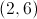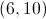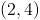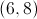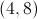Explanation:

Solve forby setting the two equations equal to one another: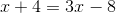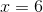Pluggingback into either equation gives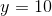.

These are the coordinates for the intersection of the two lines.

### Example Question #42 : Coordinate Geometry

Determine the greater quantity: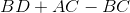or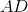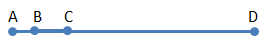The quantities are equal.The relationship cannot be determined.

The quantities are equal.

Explanation: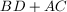is the length of the line, except that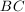is double counted. By subtracting, we get the length of the line, or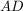.

### Example Question #41 : Coordinate Geometry

Which of the following set of points is on the line formed by the equation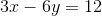?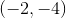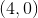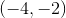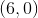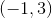Explanation:

The easy way to solve this question is to take each set of points and put it into the equation. When we do this, we find the only time the equation balances is when we use the points.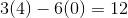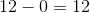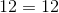For practice, try graphing the line to see which of the points fall on it.

### Example Question #6 : How To Find Out If A Point Is On A Line With An Equation

Consider the lines described by the following two equations:

4y = 3x2

3y = 4x2

Find the vertical distance between the two lines at the points where x = 6.

12

36

21

44

48

21

Explanation:

Since the vertical coordinates of each point are given by y, solve each equation for y and plug in 6 for x, as follows:Taking the difference of the resulting -values give the vertical distance between the points (6,27) and (6,48), which is 21.

### Example Question #232 : Geometry

For the line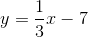Which one of these coordinates can be found on the line?

(9, 5)

(6, 5)

(6, 12)

(3, 6)

(3, 7)

(3, 6)

Explanation:

To test the coordinates, plug the x-coordinate into the line equation and solve for y.

y = 1/3x -7

Test (3,-6)

y = 1/3(3) – 7 = 1 – 7 = -6   YES!

Test (3,7)

y = 1/3(3) – 7 = 1 – 7 = -6  NO

Test (6,-12)

y = 1/3(6) – 7 = 2 – 7 = -5  NO

Test (6,5)

y = 1/3(6) – 7 = 2 – 7 = -5  NO

Test (9,5)

y = 1/3(9) – 7 = 3 – 7 = -4  NO

### Example Question #8 : How To Find Out If A Point Is On A Line With An Equation

Solve the following system of equations:

–2x + 3y = 10

2x + 5y = 6

(3, 5)

(2, 2)

(–2, 2)

(–2, –2)

(3, –2)

(–2, 2)

Explanation:

Since we have –2x and +2x in the equations, it makes sense to add the equations together to give 8y = 16 yielding y = 2.  Then we substitute y = 2 into one of the original equations to get x = –2.  So the solution to the system of equations is (–2, 2)

### Example Question #51 : Lines

Which of the following sets of coordinates are on the line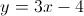?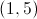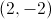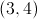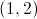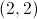Explanation:when plugged in for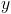andmake the linear equation true, therefore those coordinates fall on that line.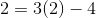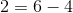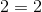Because this equation is true, the point must lie on the line. The other given answer choices do not result in true equalities.

### Example Question #10 : How To Find Out If A Point Is On A Line With An Equation

Which of the following points can be found on the line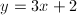?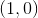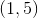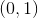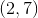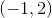Explanation:

We are looking for an ordered pair that makes the given equation true. To solve, plug in the various answer choices to find the true equality.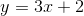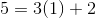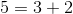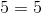Because this equality is true, we can conclude that the pointlies on this line. None of the other given answer options will result in a true equality.

Tired of practice problems?

Try live online GRE Math prep today.